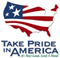# The National Flood-Frequency Program—Methods for Estimating Flood Magnitude and Frequency in rural areas New Mexico, 2000

#### U.S. Geological Survey Fact Sheet 055-00 October, 2000

This report is available in pdf 507KB.

## Introduction

Estimates of the magnitude and frequency of flood-peak discharges and flood hydrographs are used for a variety of purposes, such as for the design of bridges, culverts, and flood-control structures; and for the management and regulation of flood plains. To provide simple methods of estimating flood-peak discharges, the U.S. Geological Survey (USGS) has developed and published equations for every State, the Commonwealth of Puerto Rico, American Samoa and a number of metropolitan areas in the United States. In 1993, the USGS, in cooperation with the Federal Emergency Management Agency and the Federal Highway Administration, compiled all current USGS statewide and metropolitan area equations into a computer program, titled “The National Flood-Frequency (NFF) Program” (Jennings and others, 1994).

Since 1993, new or updated regression equations have been developed by the USGS for various areas of the Nation. These new equations have been incorporated into an updated version of the NFF Program.

This Fact Sheet describes the application of the updated NFF Program to streams that drain rural areas in New Mexico. Information on obtaining the NFF software and fact sheets for other areas of the Nation is provided at the end of this Fact Sheet.

## Overview

New Mexico is divided into eight hydrologic regions (fig. 1) on the basis of physiography, elevation, and precipitation. Waltemeyer (1996) developed regression equations for estimating peak discharges (QT), in cubic feet per second, that have recurrence intervals (T) that range from 2 to 500 years for ungaged, unregulated, rural streams in each of these regions. A ninth set of equations were developed for small watersheds that drain less than 10 square miles and that are less than 7,500 feet in mean basin elevation.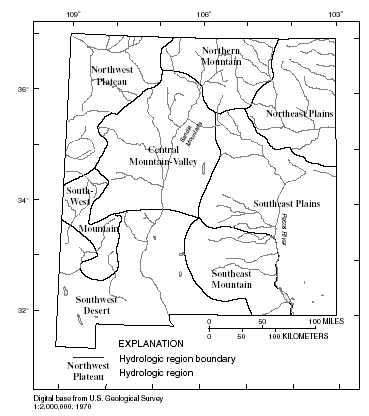Figure 1. Hydrologic regions of New Mexico.

Recurrence interval is the reciprocal of the annual exceedance probability and represents the average number of years between exceedances. For example, a flood-peak discharge with an exceedance probability of 0.01 has a recurrence interval of 100 years. This does not imply that the 100-year flood will be equaled or exceeded each 100 years, but that it will be equaled or exceeded on the average once every 100 years.

Waltemeyer (1996) provided the peak-discharge estimates (QT) and explanatory watershed variables for the 201 streamflow-gaging stations used to develop the regression equations. The peak-discharge estimates were determined from available records through 1993. An envelope curve of the relation between maximum observed peak discharge and drainage area was also provided for each region.

## Procedure

The equations are based on the inch-pound system of units, but the NFF Program will accept or report either the inch-pound or metric system of units. The explanatory watershed variables used in the regression equations shown in table 1 are as follow:

Drainage area (A), in square miles, is the total area that contributes runoff upstream of the stream site of interest, measured by planimeter using the largest scale topographic map available.

Average channel elevation (Ec), in feet above sea level [National Geodetic Vertical Datum of 1929 (NGVD of 1929)], is the average of the elevations at points 10 and 85 percent of the distance along the main channel from the location of the desired peak discharge to the drainage divide.

Mean basin elevation (E), in feet above sea level (NGVD of 1929), is the mean elevation of the drainage basin determined by the grid-sampling method. A transparent grid is placed over a topographic map on which the basin boundary is drawn. The mean elevation is determined as the average of the elevations at each grid intersection.

Maximum precipitation intensity, 24-hour duration storm, 10-year recurrence interval (I24,10), in inches, is the depth of rainfall from a 24-hour duration storm with a 10 percent chance of being exceeded in any given year at the stream site of interest, as determined by interpolation between lines of equal intensity from the National Oceanic and Atmospheric Administration (NOAA) Atlas 2 precipitation frequency map (Miller and others, 1973).

Maximum precipitation intensity, 24-hour duration storm, 25-year recurrence interval (I24,25), in inches, is the depth of rainfall from a 24-hour duration storm with a 4 percent chance of occurring in any given year at the stream site of interest, as determined by interpolation between lines of equal intensity from the NOAA Atlas 2 precipitation frequency map (Miller and others, 1973).

The regression equations and the standard errors of prediction are shown in table 1. The standard error of prediction is a measure of the accuracy of a regression equation when estimating peak-discharges for ungaged watersheds similar to those that were used to derive the regression equations. Errors in the QT estimates for about two thirds of the ungaged watersheds will be less than or equal to the given standard errors of prediction. The standard error of prediction increases appreciably when any explanatory watershed variable is near or beyond the range limits shown in table 2, or when the location of the desired peak discharge is east of the Pecos River in the Southeast Plains region or in the Sandia Mountains in the Central Mountain Valley region, where limited peak-discharge data are available.

 Regression equation Table 1. Flood-peak discharge regression equations and associated statistics for streams that drain rural areas in New Mexico (modified from Waltemeyer, 1996) [QT, peak discharge for recurrence interval T, 2 to 500 years, in cubic feet per second; A, drainage area, in square miles; E, mean basin elevation, in feet above sea level; Ec, average channel elevation, in feet above sea level; I24,10, maximum precipitation intensity, 24-hour duration storm, 10-year recurrence interval, in inches; I24,25, maximum precipitation intensity, 24-hour duration storm, 25-year recurrence interval, in inches] Q2 = 1.14 x 102A0.53 96 Q5 = 3.07 x 102A0.50 78 Q10 = 5.08 x 102A0.49 75 Q25 = 8.53 x 102A0.48 72 Q50 = 1.18 x 103A0.48 72 Q100 = 1.58 x 103A0.48 75 Q500 = 2.80 x 103A0.48 82 Q2 = 8.47 x 101A0.47 111 Q5 = 1.97 x 102A0.46 82 Q10 = 3.06 x 102A0.46 72 Q25 = 4.86 x 102A0.45 66 Q50 = 6.54 x 102A0.45 63 Q100 = 8.53 x 102A0.45 63 Q500 = 1.45 x 103A0.45 66 Q2 = 8.54 x 106A0.60(E/1,000)-5.96 36 Q5 = 7.14 x 107A0.67(E/1,000)-6.69 38 Q10 = 1.60 x 108A0.70(E/1,000)-6.94 41 Q25 = 3.04 x 108A0.75(E/1,000)-7.10 43 Q50 = 4.15 x 108A0.78(E/1,000)-7.16 46 Q100 = 5.21 x 108A0.81(E/1,000)-7.19 49 Q500 = 7.11 x 108A0.87(E/1,000)-7.20 60 Q2 = 8.17 x 101A0.51 192 Q5 = 2.36 x 102A0.54 124 Q10 = 4.07 x 102A0.55 103 Q25 = 7.21 x 102A0.57 88 Q50 = 1.04 x 103A0.58 78 Q100 = 1.43 x 103A0.59 72 Q500 = 2.72 x 103A0.62 66 Q2 = 8.54 x 102A0.83(E/1,000)-2.22I24,250.31 92 Q5 = 7.39 x 103A0.81(E/1,000)-3.01I24,250.63 82 Q10 = 2.19 x 104A0.81(E/1,000)-3.41I24,250.81 78 Q25 = 6.90 x 104A0.80(E/1,000)-3.85I24,251.03 75 Q50 = 1.44 x 105A0.80(E/1,000)-4.13I24,251.18 78 Q100 = 2.80 x 105A0.80(E/1,000)-4.40I24,251.33 82 Q500 = 1.10 x 106A0.80(E/1,000)-4.95I24,251.64 92 Q2 = 7.47 x 105A0.50(Ec/1,000)-5.28I24,101.18 103 Q5 = 2.57 x 105A0.47(Ec/1,000)-4.49I24,101.76 69 Q10 = 1.53 x 105A0.46(Ec/1,000)-4.09I24,102.06 57 Q25 = 8.89 x 104A0.44(Ec/1,000)-3.67I24,102.37 46 Q50 = 6.11 x 104A0.43(Ec/1,000)-3.38I24,102.57 43 Q100 = 4.18 x 104A0.42(Ec/1,000)-3.09I24,102.74 41 Q500 = 1.78 x 104A0.40(Ec/1,000)-2.45I24,103.03 43 Q2 = 1.28 x 102A0.46 57 Q5 = 2.46 x 102A0.48 51 Q10 = 3.45 x 102A0.49 51 Q25 = 4.91 x 102A0.50 54 Q50 = 6.15 x 102A0.51 57 Q100 = 7.51 x 10 2A0.52 60 Q500 = 1.12 x 10 3A0.55 72 Q2 = 2.58 x 107A0.19(Ec/1,000)-6.10 88 Q5 = 1.49 x 107A0.23(Ec/1,000)-5.53 85 Q10 = 1.03 x 107A0.25(Ec/1,000)-5.19 85 Q25 = 6.53 x 106A0.27(Ec/1,000)-4.80 88 Q50 = 4.69 x 106A0.29(Ec/1,000)-4.52 92 Q100 = 3.40 x 10 6A0.30(Ec/1,000)-4.25 96 Q500 = 1.66 x 10 6A0.32(Ec/1,000)-3.68 116 Q2 = 1.07 x 102A0.39 120 Q5 = 2.43 x 102A0.42 88 Q10 = 3.74 x 102A0.43 75 Q25 = 5.91 x 102A0.44 69 Q50 = 7.92 x 102A0.45 66 Q100 = 1.03 x 103A0.46 63 Q500 = 1.73 x 103A0.47 63

 Hydrologic study region Drainage area, in square miles Average channel elevation, Mean basin elevation, in feet above sea level1 in feet above sea level1 Maximum 24-hour precipitation intensity for indicated recurrence interval in inches Table 2. Range of explanatory variables for which regression equations are applicable [--, data not statistically significant] Northeast plains 0.36–2,060 -- -- -- -- Northwest plateau .17–925 -- -- -- -- Southeast mountain 3.08–947 -- 5,440– 9,060 -- -- Southeast plains .16–689 -- -- -- -- Northern mountain .63–2,850 -- 7,810– 11,400 -- 2.00–4.45 Central mountain valley .16–3,660 5,310– 9,280 -- 2.15–3.00 -- Southwest desert .20–2,830 -- -- -- -- Southwest mountain 2.12–426 6,160– 8,980 -- -- -- Small basins statewide .20–10.0 -- -- -- -- 1NGVD of 1929.

## Improving Estimates With Gaged Data

Waltemeyer (1996) developed weighting techniques to improve estimates of peak discharge at gaged locations by combining the estimates derived from analysis of systematic gage records with estimates derived from the regression equations.

The weights of these two independent estimates are based on the length of the gage record (in years) and the equivalent years of record of the applicable regression equation. The weighted estimate of peak discharge for the gaged site is computed as: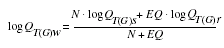where

 QT(G)w is the weighted estimate of peak discharge, QT, for recurrence interval T at the gaged location, QT(G)s is the estimate of QT derived from analysis of the systematic gage records, QT(G)r is the estimate of QT derived from application of the appropriate regression equation in table 1, N is the number of years in the gage record used to compute QT(G)s, and EQ is the equivalent years of record.

The accuracy of the weighted discharge estimate, in equivalent years of record, is equal to N + EQ. The NFF Program performs these computations.

## Ungaged Sites Near Gaged Sites on the Same Stream

Waltemeyer (1996) also showed how the weighted estimate for peak discharge at a gaged site can be used to improve estimates of peak discharge of an ungaged site on the same stream that has a drainage area that is between 50 and 150 percent of the drainage area of the gaged site. The weighted estimate for the ungaged site is computed as: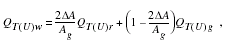where

 QT(U)w is the weighted estimate of discharge QT for recurrence interval T at the ungaged site, ΔA is the absolute value of the difference between the drainage areas of the gaged site, (Ag), and the ungaged site, (Au), |Ag - Au|, QT(U)r is the peak-flow estimate for recurrence interval T at the ungaged site derived from the applicable regional regression equation (table 1), QT(U)g is the peak-flow estimate for recurrence interval T at the ungaged site derived from the weighted estimate of peak discharge at the gaged site, QT(G)w, by adjusting for the effect of the difference in drainage area between the gaged site and the ungaged site. QT(U)g is computed as: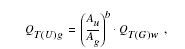where b is the exponent of drainage area from the appropriate regression equation (table 1).

This weighting method can be used to improve the estimate at the ungaged site when the drainage area at the ungaged site is within 50–150 percent of the drainage area of the gaged site. Otherwise, the estimate at the ungaged site should be based only on the appropriate regression equation from table 1.

## Sites in Transition Zones

When the drainage area of the site of interest is in more than one of the regions, a weighted estimate of the peak discharge should be computed. The equations for the appropriate regions should be applied independently using basinwide estimates of the required explanatory variables. The weighted estimate is then computed by multiplying each regional estimate against the fraction of the drainage area in that region and summing the products. The NFF Program provides an algorithm for this computation.

## References

Jennings, M.E., Thomas, W.O., Jr., and Riggs, H.C., comps., 1994, Nationwide summary of U.S. Geological Survey regional regression equations for estimating magnitude and frequency of floods for ungaged sites, 1993: U.S. Geological Survey Water-Resources Investigations Report 94–4002, 196 p.

Miller, J.F., Frederick, R.H., and Tracey, R.J., 1973, Precipitation-frequency atlas of the western United States, volume IV—New Mexico: National Oceanic and Atmospheric Administration NOAA Atlas 2, 43 p.

Waltemeyer, S.D., 1996, Analysis of the magnitude and frequency of peak discharge and maximum observed peak discharge in New Mexico: U.S. Geological Survey Water-Resources Investigations Report 96–4112, 79 p.

U.S. Geological Survey

Office of Surface Water

415 National Center

Reston, Virginia 20192

(703) 648-5301

USGS hydrologic analysis software is available for electronic retrieval through the World Wide Web (WWW) at:
http://water.usgs.gov/software/
and through anonymous File Transfer Protocol (FTP) from water.usgs.gov (directory: /pub/software). The WWW page and FTP directory from which the National Flood-Frequency software and user documentation can be retrieved are http://water.usgs.gov/software/nff.html and /pub/software/surface_water/nff, respectively.

Additional earth science information is available from the USGS through the WWW at http://www.usgs.gov/ or by calling 1-888-ASK-USGS.

 AccessibilityFOIAPrivacyPolicies and Notices U.S. Department of the Interior, U.S. Geological Survey Persistent URL: http://pubsdata.usgs.gov/pubs/fs/fs-055-00/ Page Contact Information: USGS Publishing Network Last modified: Tuesday, November 29 2016, 05:53:33 PM